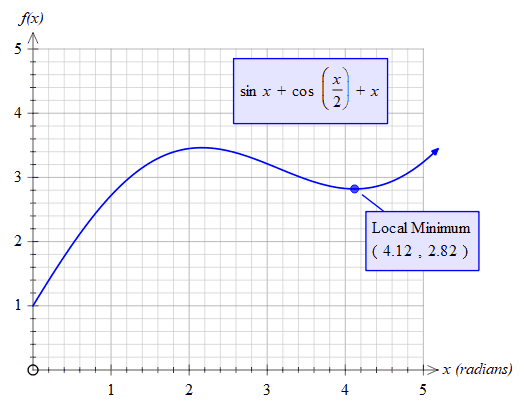﻿ fnMin

# fnMin

Top  Previous  Next
 fnMin(function, low, high)   Finds the x value which produces the lowest y value between low and high. For example:fnmin(sinx+cos(x/2)+x,2,5) = 4.12 fnmin(sinx+cos(x/2)+x,0,1) = 0   To find the y value of the local maximum, use the fnminval function